When preparing samples and experiments in the lab, you are faced with also having to prepare solutions in molar or normal concentrations. In other cases, you have to make solutions from hydrated compounds.

To do this, you have to recall your stoichiometry lessons. And if you’re new to stoichiometry, this could feel a little overwhelming. It’s during these moments, you might wish you had a quick tool to refresh all those concepts and quickly calculate the solutions you need.

Fear not, because in this article, we will refresh you about the terms molar, normal, and you will learn how to prepare molar and normal solutions.

Finally, we will provide a step-by-step procedure to help you prepare solutions from hydrated compounds.

## Molarity vs. normality

Molarity is defined as the number of moles of solute per liter of solution. It is represented as

M = moles/liter

Normality, also known as equivalent concentration, is defined as the number of equivalent weights per unit volume, generally a liter of solution.

An equivalent weight is the ratio of a chemical species' formula weight, FW, to the number of its equivalents, n.

For instance:

Equivalent weight H2SO4 =

[1g * 2 equivalents of H] +

[32.06g * 1 equivalents of S)] +

[16g * 4 equivalents of O] = 98 g

Here the number of equivalent weights = 98 g

Then, the normality is represented as

N = number of equivalent weights/liter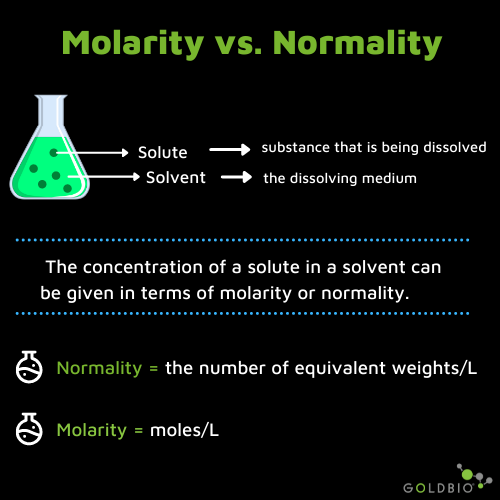The normality can be changed to molarity using the following formula.

N= M*n, where n is the valence. The valence is the number of ions that can be displaced.

For example, HCL has only one hydrogen which can displace, so, the valence in HCL is 1 while in H2SO4 is 2.

Therefore, in some cases, the molarity of some bases and acids can be the same, when the valence is equal to one. In other cases, it can differ and it depends on the valence.

For instance, the molarity and normality of HCL is the same. On the other hand, to calculate the normality of H2SO4, the molarity must be dived by two, due to its valence of = two.

## How to prepare a molar solution

To prepare a molar solution, you need the molecular weight of your compound, the desired final volume, and the desired molarity.

Use the following formula to calculate the amount of compound to get the specific molarity.Example: Prepare 100 mL of 2M CaCl2.

Desired concentration = 2M = 2 moles/liter

Molecular weight CaCl2 = 110.9848 g/mol

Desired final volume = 100 mLIn this case, you need to add 22.196 g of CaCl2 and dilute to 100 mL to prepare a 2M solution.

If you’re new to stoichiometry, one of the challenges is how you set up the equation. How do you know what numbers go on the top and which go on the bottom of the fraction bars?

One of the best tips, is to set the equation up so your units cancel out. Pay attention to the animation below as it shows exactly how this work. The units that are not canceled out are your remaining units to use in your solution.

Stoichiometry Demo## How to prepare a normal solution

To prepare a normal solution, you need the number of equivalent weights of your compound, the desired final volume, the desired normality, and the valence. The valence is the number of ions that can be displaced.

In the next example, I will show you how to calculate the equivalent weight and find the valence.

Use the formula below to calculate the amount of compound to get the specific normality.Example: Prepare 100 mL of 1N H2SO4.

Desired concentration = 1 Normal. A concentration of 1N is the same as having 1 number of equivalent weight/liters

Gram-equivalent weight H2SO4 = [1*(2) of H] + [32.06 of S] + [16*(4) of O] = 98 g

Valence of H2SO4 = 2, because there are 2 hydrogen ions (H2) that could be displaced

Desired final volume = 100 mL = 0.1 LPlugging the values for the above formula we have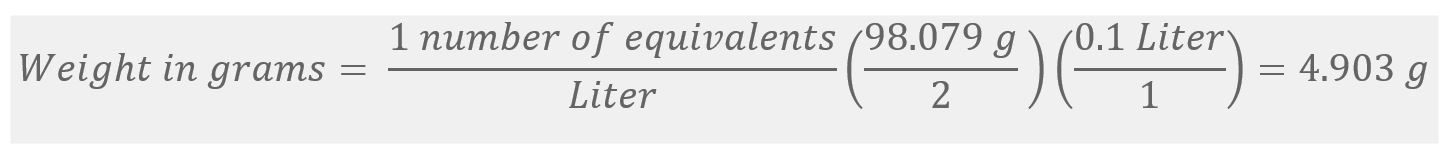Where, liter with liter units is canceled out, and the number of equivalents do not have a unit, so we only get with grams as final unit.

In this example, you need to add 4.903 g of H2SO4 and dilute it to 100 mL to prepare a 1N solution.

### Step-by-step molar/normal solution preparation

• Calculate the amount of the compound you need to add with the above formula.
• Put about 80% of the water volume into a beaker.
• Add the calculated amount of your compound and mix well until dissolving.
• Transfer the solution to a graduated cylinder and add water to complete it until the desired volume is reached.

## Standard solutions prepared in the lab

Next, I will give you the amount you need to prepare standard solutions in the lab.

### 1M NaOH

To prepare 1M from NaOH pellets consider:

Molecular weight NaOH = 40g/mol

Desired final volume= 100 mL = 0.1L

Molarity = 1M = 1 mol/liter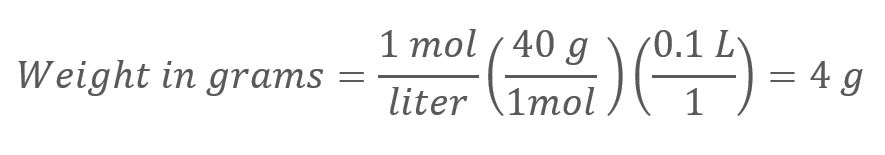Then, put 50 ml of water into a mixing vessel, add 4 g of NaOH pellets, and dissolve it by mixing. Finally, fill the vessel up to 100 ml with water using a graduated cylinder.

Note: Always wear gloves, goggles, and a lab coat when working with concentrated NaOH because it is a very strong base that can cause injury upon contact.

### 1M HCl

Preparing HCl is slightly different from NaOH because HCl is usually in liquid form. Typically, HCl comes in a concentration of 37%.

To prepare 100 ml of 1M from liquid HCl at 37% consider:

• Density of HCl at 37% = 1.19 kg/L. It is associated with the density relative of HCL to the density of water.
• Molecular weight HCl = 36.5 g/mol
• Desired final volume= 100 mL = 0.1L
• Molarity = 1M = 1 mol/liter

Because HCl is in liquid form, you need to convert the percentage to molarity. The mass fraction is expressed as mass percentage, and it is expressed as % weight to weight or %w/w.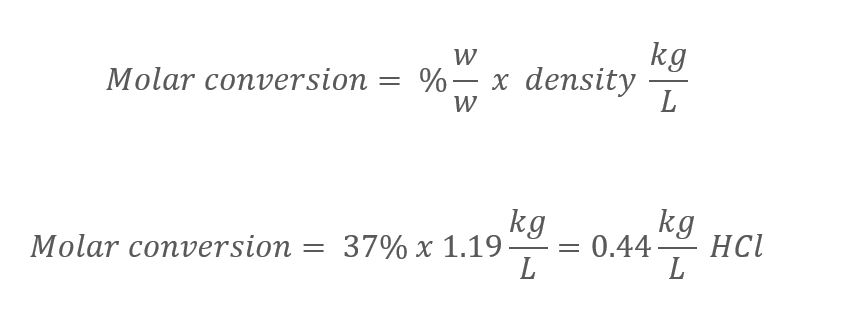Then, use this value to calculate the molarity of HCL. Then, use this value to calculate the molarity of HCL.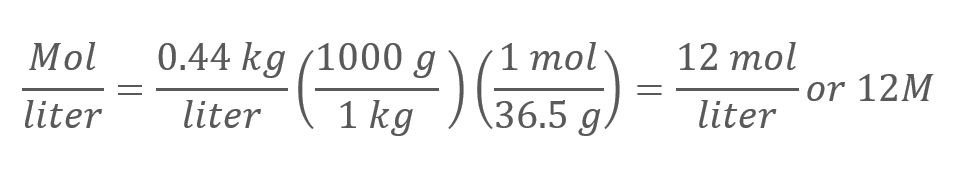To prepare 1M of HCl from liquid HCl 37%, calculateOr

C1 * V1 = C2 * V2

Where,

C1 = 12M (or the beginning concentration)

V1 = volume to calculate

C2 = 1M (desired concentration)

V2 = 100 mL or 0.1 L (desired final volume)

From the above equation, we calculate V1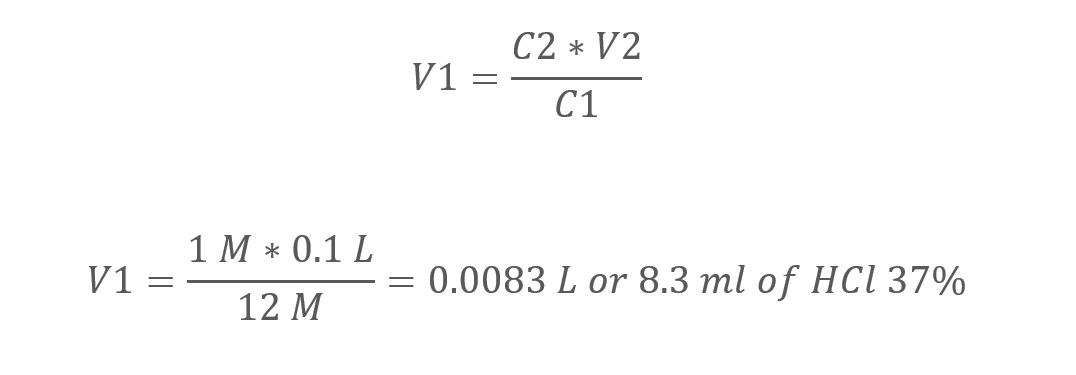Then, put 50 ml of water into a mixing vessel, add 8.3 ml of HCl carefully, and once it is dissolved, fill it up to 100 ml with water using a graduated cylinder.

Note: Always wear gloves, goggles, and a lab coat when working with concentrated HCl, because it is a very strong acid that will burn your skin instantly upon contact.

## Make a solution from hydrated compound

When you are following a reported culture media recipe, you will most likely face a situation where you need to prepare a solution from a reagent without water, an anhydrous reagent.

In this case, you have the same reagent but with seven more water molecules, a heptahydrate reagent.

How much do you add into your reagent's solution to prepare the same concentration as the reference? Below, I will detail how to do this.

### 1.Identify if the form of your reagent that matches what is reported in your protocol.

It is essential to know some reagents change their form (monohydrate, tetrahydrate, anhydrous) according to the water content.

For instance, ferrous chloride (FeCl2) can have two water molecules (dihydrate) or four water molecules (tetrahydrate). The water molecules are represented as an integer before the water formula H2O.

In the first example, this reagent's formula is FeCl2 2H2O. In the second example, the reagent's formula is FeCl2 4H2O.

Below is a table where you can find common salts used in plant tissue culture media with different forms regarding the water content.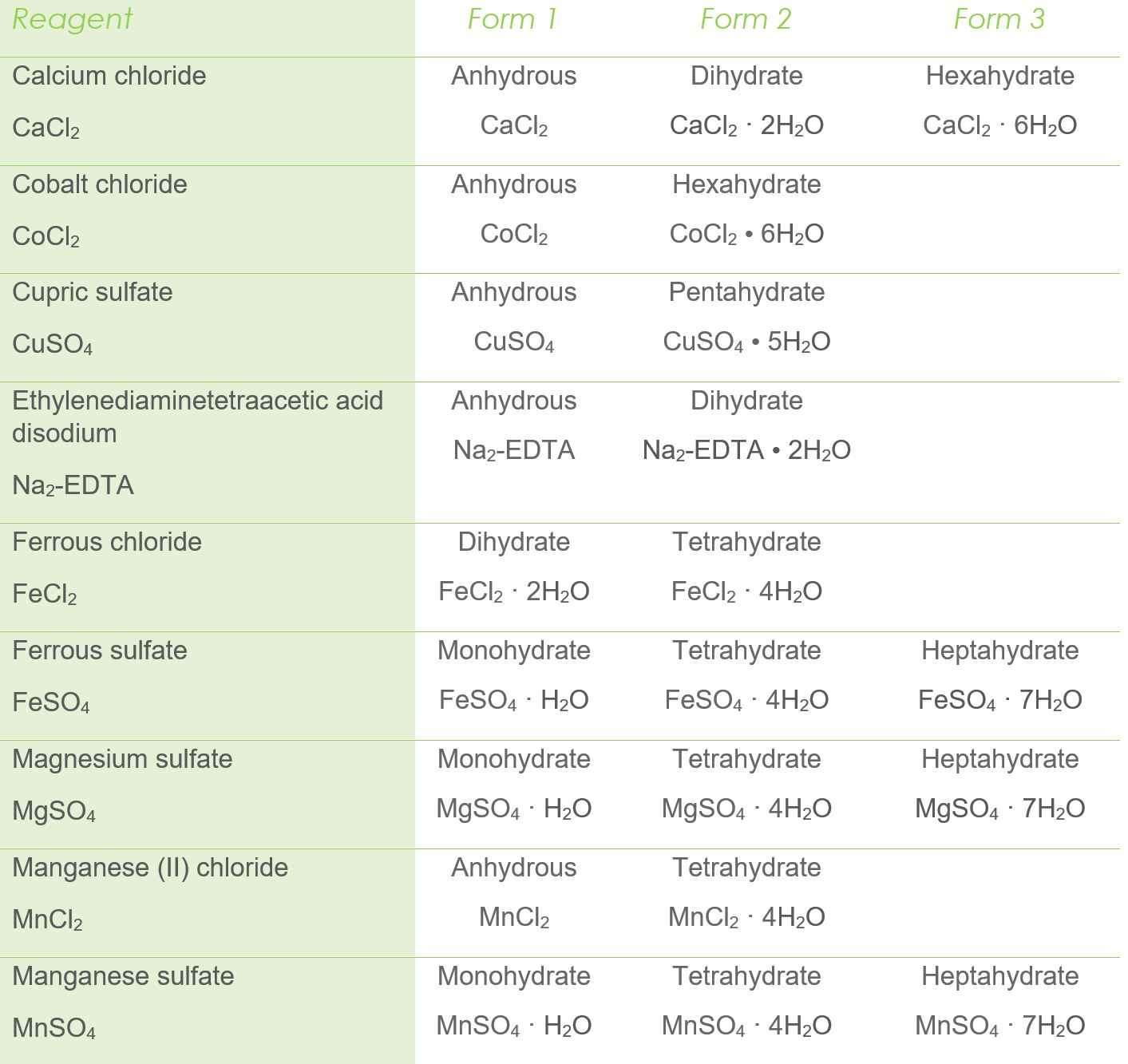### 2.Calculate the amount of reagent to add

As you already know, the plant tissue culture reagents can show different forms according to the water content.

Once you identified what is needed and what you have, calculate the amount of your reagent to add to match the same concentration as the reported recipe.

Let's say you are asked to prepare a solution with

3.33 g/L of MnSO4 • H2O

However, you have MnSO4 4H2O as a reagent.

How much do you add of MnSO4 4H2O to get 3.33g/L?

To calculate the amount to add, you need the molecular weight of the salt on an anhydrous basis.

Consider that one molecule of H2O = 18g, so

MnSO4 = 151.00g (anhydrous basis)

MnSO4 • H2O = 151.00g + (18g) = 169 g

MnSO4 • 4H2O = 151.00g + (18g x 4) = 223 g

Next, this formula will help you to easily calculate how much you should add considering the water content.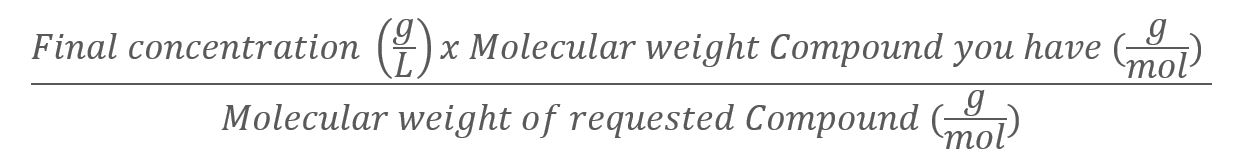Thus, for the above example, the formula isThen, if you follow a protocol that requires you to prepare 3.33 g/L of MnSO4 • H2O, but there is only MnSO44H2O in your lab, you need to add 4.39g/L MnSO44H2O to match the initial concentration.

## References

Choudhary, A. (2008). Preparation of Molar and Normal Solutions. Pharmaceutical Guidelines.

OpenWetWare. (2018). Hydrochloric acid. OpenWetWare.

Varsity Tutors. (2021). Molarity, Molality, Normality. Varsity Tutors.